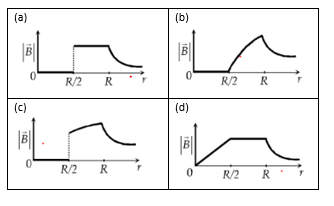# Multiple Choice questions on Magnetic field and magnetic effects of current for JEE Main and Advanced

Question 1
Find the force on the straight conductor of length .30 m carrying a current of 5 .0 A in the – k direction where the magnetic Field is given by B=3.5 ×10-3 (i - j) T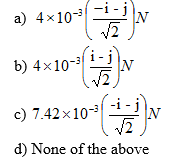Question 2
A current sheet having current K A/m is placed at in the x-y plane at z=0.The direction of current is -j
Find the Magnetic Field at on the z axis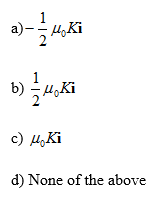Question 3
A current sheet where current per unit length is 6.0 A/m and direction is towards +i is placed at z=0 in the x-y plane. A current wire is located at y=0 and z=5 m. It is placed along the x axis. Find the current and its direction if Magnetic field is zero at the point P whose location is (0, 0, 2.5) m
(a) 47 A, i
(b) 47.1 A, -i
(c) 50 A, i
(d) 50 A, -i

Questions 4
A charged particle whose mass is 19.9 ×10-27 kg and charge is 1.6×10-19 C moves with a speed of 3×105 m/s at right angle to a magnetic field of .75 T.
What is the force acting on the charge, centripetal acceleration and radius of the circle in which charged particle moves
(a) 3.6×10-14 N,1.81×1012 m/s2, 49.7×10-3 m
(b) 3.0×10-14 N,1.81×1012 m/s2, 49.7×10-3 m
(c) 3.0×10-14 N,1.8×1012 m/s2, 49.7×10-3 mm
(d) None of the above

Question 5
Which of the following is true?
(a) Magnetic field exerts force only a moving charge.
(b) Electric field exerts force on both stationary and moving charge
(c) Magnetic field exerts force on charge moving parallel to the direction of the field
(d) All the above

Question 6
Four Particles P1(proton), P2(electron), P3(alpha), P4(neutron) enters a region of constant magnetic field with same velocities. The magnetic field is perpendicular to the velocity
Match the following
Column A
(a) what all particle will not be deflected by the Magnetic field
(b) What all particles will be deflected by the Magnetic Field?
(c) Which particle move in a circular path of maximum radius
(d) Which particle will experience maximum Force?
Column B
(p) P1
(q) P2
(r) P3
(s) P4

Question 7
The force experienced by a particle of charge q moving with a velocity v in a uniform magnetic field B is given by
F=q(vXB)
Statement I: The vectors F and v are perpendicular to each other
Statement II: The vectors v and B can have any angle between them
Statement III: The vectors F and B are perpendicular to each other
(a) All the statements are true
(b) Statement I and II are true only
(c) Statement I and III are true only
(d) Statement II and III are true only

Question 8
A long wire is bent as shown in figure. Find the magnitude and direction of the magnetic field at the center of the circular part if a current of I amp is passed through the wire
The different parts of the wire do not touch each other at Q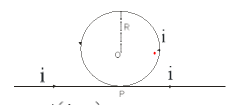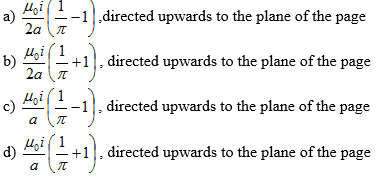Question 9
A non-conducting thin disc of Diameter D charged uniformly over one side with surface density$\sigma$ rotates about its axis with an angular velocity $\omega$
What is the magnetic field at the center of the disc?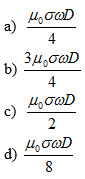Question 10
A charged particle moving with constant velocity passes through a space without any change in velocity
Electric Field in the region is given by the
E=a1i + a2 j + a3 k
Magnetic Field in the region is given by the
B=b1i + b2 j + b3 k
Where a1, a2, a3, b1, b2, b3 are constant
Which of the following can be true about the region?
(a) a1=a2=a3=b1=b2=b3 =0
(b) a1=a2=a3 =0, b1≠0 b2≠0 b3≠0
(c) a1≠0 a2≠0 a3≠0, b1=b2=b3 =0
(d) a1≠0 a2≠0 a3≠0 b1≠0 b2≠0 b3≠0

Question 11
A charged particle of mass m and charge q moves with a constant velocity along the positive x direction
v=a i.
it enters a region of Magnetic field which is directed towards positive z direction from x=a which is given by
B = b k
Find the initial acceleration of the particle
(a) a=(-qab/m) j
(b) a=(-qab/m) j
(c) a=(-qa/mb) j
(d) none of these

Question 12
Find the radius of the circular path which the particle moves
(a) mb/qa
(b) ma/q
(c) mab/q
(d) None of these

Question 13
Which of the following is true about the motion of the particle?
(a) Force will always be perpendicular to the velocity
(b) The magnitude of the velocity remains constant
(c) velocity vector and magnetic field vector remains perpendicular to each other during the motion
(d) KE of the particle remains constants

Question 14
The frequency of the rotation
(a) depends on the value a
(b) depends on the value b
(c) depends on the value a and b both
(d) Does not depend on a and b both

Question 15
Four circular coil( A,B,C,D) having radius r,2r,3r,4r having numbers of turns 4n,3n,2n,n and carrying the same current are there.
Match the Column
Column X
(P) which coil will produce maximum Magnetic induction at the center
(Q) which coil will produce minimum Magnetic induction at the center
(R) Which coil has maximum magnetic moment per turn
(S) Which coil has minimum magnetic moment per turn
Column Y
(E) A
(F) B
(G) C
(H) D

Question 16
An infinite long thin cylinder shell of radius R is carrying current I along the axial direction.
Which of the following is true?
(a) B =0 for r < R
(b) B =k/r for r > R
(c) B=kr for r > R
(d) none of the above
Where $k= \frac {\mu _0 I}{2\pi }$

## Paragraph Based Questions

(A) A particle of mass m and electric charge q is in a Magnetic field which varies as
$\mathbf{B}=\frac {\mu _0}{4 \pi}\frac {k}{r^3} \mathbf{r}$
Where k is constant and r is the radius vector from origin

Question 17
If the particle has velocity vector v, position vector r .Find the acceleration of the particle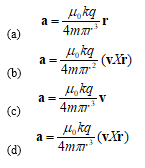Question 18
which of the following is true
(a) $\mathbf{a}.\mathbf{v}=0$
(b) Speed v=|v| is constant in the motion
(c) $\mathbf{a}.\mathbf{r}=0$
(d) $m(\mathbf{r} \times \mathbf{v}) -q \mathbf{B} r^2$ Vector quantity is a constant of the motion

(B) A charge particle Q of mass m at rest is released from origin at t=0 in the xyz plane.
A constant magnetic field exists in the plane
$\mathbf{B}= B\mathbf{i}$
A constant electric field also exists in the plane
$\mathbf{E}= E\mathbf{k}$
Hint
It is given equation of following form gives following result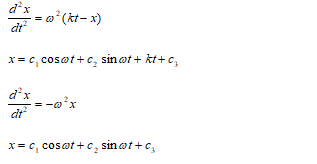Question 19
The force acting on the particle at any point of time will be of the form
$\mathbf{F}= a\mathbf{i} + b\mathbf{j} + c\mathbf{k}$
(a) a is zero constant through out the motion
(b) b varies with the motion and it becomes zero at some points
(c) c varies with the motion and it never becomes zero
(d) b varies with the motion and it never become zero
Question 20
Find velocity of the particle as a function of time t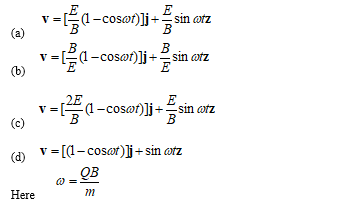Question 21
Find position vector of the particle as a function of time t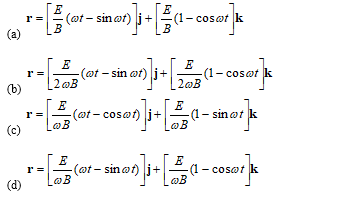Question 22
If the particle starts with the velocity at t=0
$\mathbf{v_0}=\frac {E}{B} \mathbf{j}$
Find out which of the following is true
(a) The particle moves in a straight line along y axis
(b) The velocity of the particle does not change during the motion
(c) The velocity vector will vary like
$\mathbf{v}=\frac {E}{B} \mathbf{j} + \frac {E}{B} \sin \omega t \mathbf{k}$ where $\omega =\frac {QB}{m}$
(d) The particle moves like a cycloid

## Multiple Choice Questions

Question 23
A parabolic section of wire is located in the XY plane and caries current i. A uniform magnetic field exists throughout the plane and it is given by
$\mathbf{B}=B_0( \mathbf{i} + \mathbf{j})$
The equation of the parabola is
$y=4x^2$
Calculate the total force on the wire between origin and the point P where x=1
(a) $(4IB_0) \mathbf{k}$
(b) $(-4IB_0) \mathbf{k}$
(c) $(3IB_0) \mathbf{k}$
(d) $(-3IB_0) \mathbf{k}$

Question 24
A uniformly positively charged disk of mass $M_0$ whose total charge has magnitude Q and whose radius is R rotates with constant angular velocity of magnitude $\omega$
Which one of the following is true
(a) Angular momentum of the disk is $\frac {1}{2}M_0 R^2 \omega$
(b) The ratio of Magnetic moment and angular momentum is $\frac {Q}{2M_0}$
(c) The angular momentum and Magnetic moment direction are antiparallel
(d) None of these

Question 25
The current density in the wire varies with r according to the formula
$J=r^2$ for r< a
$J=0$ for r > a
(a) The magnetic field is proportional to 1/r for r > a
(b) The magnetic field at r=a is given by
$B = \frac {\mu _0 a^3}{4}$
(c) The magnetic field is proportional to $r^4$ for r < a
(d) The magnetic field is proportional to $r^3$ for r < a

Question 26
A proton is fixed at the origin and electron revolves about it in a circular path of radius R.
$M_p$ -> Mass of proton
$M_e$ -> Mass of electron
q -> Charge on electron and proton
Find the magnetic field at the proton
(a) $B= \frac {\mu _0 q^2}{4 \pi R^2 \sqrt {4 \pi \epsilon _0 M_e R}}$
(b) $B= \frac {\mu _0 q^2}{2 \pi R^2 \sqrt {4 \pi \epsilon _0 M_e R}}$
(c) $B= \frac {\mu _0 q^2}{2 \pi R^2 \sqrt {4 \pi M_e R}}$
(d) $B= \frac {\mu _0 q^2}{8 \pi R^2 \sqrt {4 \pi \epsilon _0 M_e R}}$

## Paragraph Based Questions

(C) A positive charge particle of mass m and charge q is released from rest at x =-a ,y=0 and z=0 in the XYZ plane.
Electric and magnetic field exists in the XYZ plane as per below functions
$\mathbf{E}= E_0 \mathbf{i}$ for $x \leq 0$
$\mathbf{B}= -B_0 \mathbf{k}$ for $x \geq 0$

Question 27
Find the velocity of the particle when it reaches origin
(a) $v= \sqrt { \frac {2qE_0a}{m} }$
(b) $v= \sqrt { \frac {2qB_0a}{m} }$
(c) $v= \sqrt { \frac {2qE_0a}{B_0m} }$
(d) $v= \sqrt { \frac {2qB_0a}{E_0m} }$

Question 28
Find the coordinates of particle when it re-enters the electric field region
(a) $x = \sqrt { \frac {8mE_0 a}{qB_0^2}}$ ,$y=0$ ,$z=0$
(b) $x = 0$ ,$y=0$,$z=\sqrt { \frac {8mE_0 a}{qB_0^2}}$
(c) $x = \sqrt { \frac {2mE_0 a}{qB_0^2}}$ ,$y=\sqrt { \frac {8mE_0 a}{qB_0^2}}$ ,$z=0$
(d) $x = 0$ ,$y=\sqrt { \frac {8mE_0 a}{qB_0^2}}$ ,$z=0$

## Match the Column

Question 29
M is defined as
$M= \frac {B}{\mu _0}$
Column A
(P) M at the center of square loop which carries a steady current I and let a be the distance from the center to the side
(Q) M at the center of regular 6 sided polygon which carries a steady current I and let a be the distance from the center to the side
(R) M at the center of n sided polygon polygon which carries a steady current I and let a be the distance from the center to the side
(S) M at the center of n sided polygon polygon in the limit n-> 8 which carries a steady current I and let a be the distance from the center to the side
Column B
(A) $\frac {I}{2a}$
(B) $\frac {I \sqrt 2}{ \pi a}$
(C) $\frac {3I}{ 2\pi a}$
(D) $\frac {nI}{ 2\pi a} \sin \frac {\pi}{n}$
(E) $\frac {I}{ 2\pi a} \sin \frac {\pi}{n}$

Question 30
A long straight wire along the z axis carries a current I in negative x direction. The magnetic field vector B at a point having coordinates (x,y) in the z=0 plane is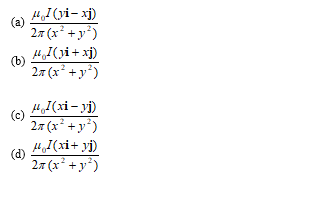Question 31
A thick slab extending from z=-b to z=+b carries a uniform current density K and direction of current is positive x axis. Which of the following is true?
(a) Magnetic field points in negative y axis for z > 0
(b) Magnetic field points in negative y axis for z < 0
(c) Magnetic field points in positive y axis for z > 0
(d) Magnetic field points in positive y axis for z < 0

Question 32
Two coaxial plane coil of radius r and separated by the distance r. The current I flows in same direction in both the coil
Which of the following statement is true?
(a) The magnetic Field on the axis at a point midway between the center is $\frac{8\mu_0I}{5r\sqrt5}$
(b) the magnetic field due to one coil on the axis decrease as the distance increases
(c) The magnetic field between the coil is relatively uniform between the coils.
(d) None of these

Question 33
Two long parallel wires are at a distance 2d apart. They carry steady equal currents flowing out of the plane of the paper, as shown. The variation of the magnetic field B along the line XX' is given by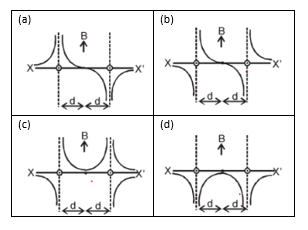Question 34
A current carrying loop is placed in a uniform magnetic field in four different orientations I, II, III and IV as shown in figure. Arrange them in decreasing order of potential energy.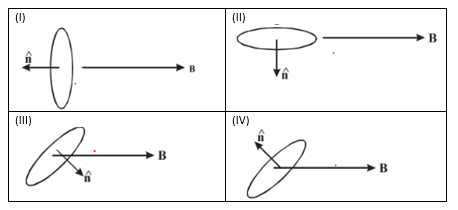(a) I > III > II > IV
(b) I > IV > II > III
(c) I > II > III > IV
(d) III > IV > I > II

Question 35
In a cyclotron, a charged particle
(a) undergoes acceleration all the time.
(b) speeds up between the dees because of the magnetic field.
(c) speeds up in a dee.
(d) slows down within a dee and speeds up between dees.

Question 36
An infinitely long hollow conducting cylinder with inner radius r/2 and outer radius R carries a uniform current density along its length . The magnitude of the magnetic field ,|B| as a function of the radial distance r from the axis is best represented by